# Three Dimensional Geometry Class 12 NCERT Solutions

NCERT Solutions Class 12 Mathematics Chapter 11 Three Dimensional Geometry Download In Pdf

## In this pdf file you can see answers of following Questions

### EXERCISE 11.1

Question 1. If a line makes angles 90°, 135°, 45° with the x, y and z-axes respectively, find its direction cosines.

Question 2. Find the direction cosines of a line which makes equal angles with the coordinate axes.

Question 3. If a line has the direction ratios –18, 12, – 4, then what are its direction cosines ?

Question 4. Show that the points (2, 3, 4), (– 1, – 2, 1), (5, 8, 7) are collinear.

Question 5. Find the direction cosines of the sides of the triangle whose vertices are (3, 5, – 4), (– 1, 1, 2) and (– 5, – 5, – 2).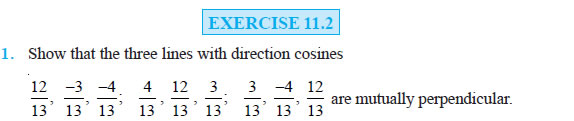Question 2. Show that the line through the points (1, – 1, 2), (3, 4, – 2) is perpendicular to the line through the points (0, 3, 2) and (3, 5, 6).

Question 3. Show that the line through the points (4, 7, 8), (2, 3, 4) is parallel to the line through the points (– 1, – 2, 1), (1, 2, 5).

Question 4. Find the equation of the line which passes through the point (1, 2, 3) and is parallel to the vector 3 iˆ + 2 ˆj −2 kˆ .

Question 5. Find the equation of the line in vector and in cartesian form that passes through the point with position vector 2 iˆ− j + 4 kˆ and is in the direction iˆ + 2 ˆj − kˆ .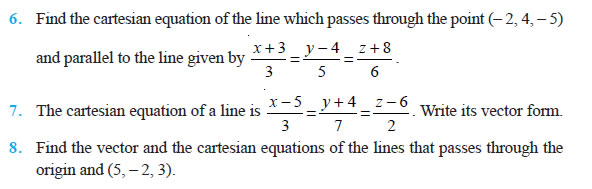Question 9. Find the vector and the cartesian equations of the line that passes through the points (3, – 2, – 5), (3, – 2, 6).

Question 10. Find the angle between the following pairs of lines: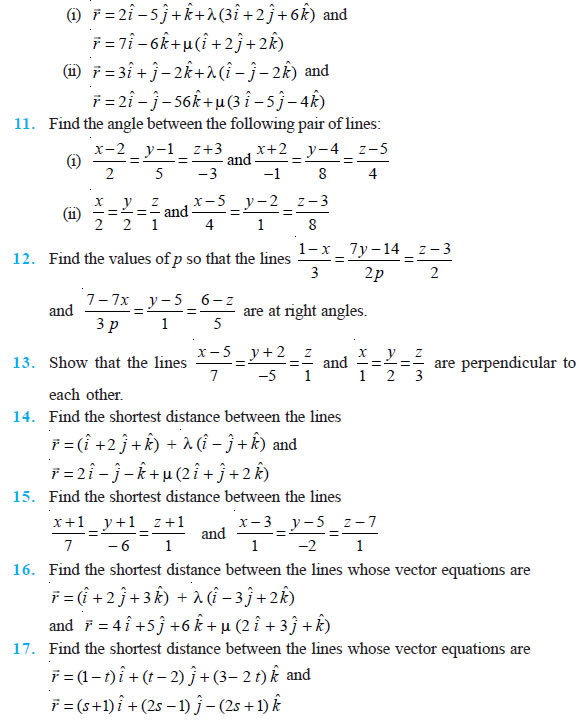### EXERCISE 11.3

Question 1. In each of the following cases, determine the direction cosines of the normal to the plane and the distance from the origin.
(a) z = 2
(b) x + y + z = 1
(c) 2x + 3y – z = 5
(d) 5y + 8 = 0

Question 2. Find the vector equation of a plane which is at a distance of 7 units from the origin and normal to the vector 3 iˆ + 5 ˆj − 6 kˆ.

Question 3. Find the Cartesian equation of the following planes:
(a) r (iˆ + ˆj − kˆ) = 2 
(b) r (2iˆ +3 ˆj − 4kˆ) = 1 
(c) r [(s − 2t) iˆ + (3 − t) ˆj +(2 s +t ) kˆ] = 15 

Question 4. In the following cases, find the coordinates of the foot of the perpendicular drawn from the origin.
(a) 2x + 3y + 4z – 12 = 0 (b) 3y + 4z – 6 = 0
(c) x + y + z = 1 (d) 5y + 8 = 0

Question 5. Find the vector and cartesian equations of the planes
(a) that passes through the point (1, 0, – 2) and the normal to the plane is iˆ + ˆj − kˆ.
(b) that passes through the point (1,4, 6) and the normal vector to the plane is iˆ−2 ˆj + kˆ.

Question 6. Find the equations of the planes that passes through three points.
(a) (1, 1, – 1), (6, 4, – 5), (– 4, – 2, 3)
(b) (1, 1, 0), (1, 2, 1), (– 2, 2, – 1)

Question 7. Find the intercepts cut off by the plane 2x + y – z = 5.

Question 8. Find the equation of the plane with intercept 3 on the y-axis and parallel to ZOX plane.

Question 9. Find the equation of the plane through the intersection of the planes 3x – y + 2z – 4 = 0 and x + y + z – 2 = 0 and the point (2, 2, 1).

Question 10. Find the vector equation of the plane passing through the intersection of the planes r .(2 iˆ + 2 ˆj − 3 kˆ ) = 7 , r .(2 iˆ + 5 ˆj + 3 kˆ ) = 9 and through the point (2, 1, 3).

Question 11. Find the equation of the plane through the line of intersection of the planes x + y + z = 1 and 2x + 3y + 4z = 5 which is perpendicular to the plane x – y + z = 0.

Question 12. Find the angle between the planes whose vector equations are r (2 iˆ + 2 ˆj − 3 kˆ) = 5 and r (3 iˆ − 3 ˆj + 5 kˆ) = 3 .

Question 13. In the following cases, determine whether the given planes are parallel or perpendicular, and in case they are neither, find the angles between them.
(a) 7x + 5y + 6z + 30 = 0 and 3x – y – 10z + 4 = 0
(b) 2x + y + 3z – 2 = 0 and x – 2y + 5 = 0
(c) 2x – 2y + 4z + 5 = 0 and 3x – 3y + 6z – 1 = 0
(d) 2x – y + 3z – 1 = 0 and 2x – y + 3z + 3 = 0
(e) 4x + 8y + z – 8 = 0 and y + z – 4 = 0

Question 14. In the following cases, find the distance of each of the given points from the corresponding given plane.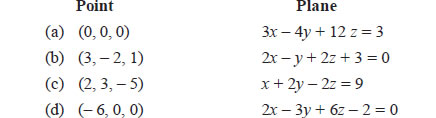### Miscellaneous Exercise on Chapter 11

Question 1. Show that the line joining the origin to the point (2, 1, 1) is perpendicular to the line determined by the points (3, 5, – 1), (4, 3, – 1).

Question 2. If l1, m1, n1 and l2, m2, n2 are the direction cosines of two mutually perpendicular lines, show that the direction cosines of the line perpendicular to both of these are 1 2 2 1 1 2 2 1 1 2 2 1 m n − m n , n l − n l , l m − l m

Question 3. Find the angle between the lines whose direction ratios are a, b, c and b – c, c – a, a – b.

Question 4. Find the equation of a line parallel to x-axis and passing through the origin.

Question 5. If the coordinates of the points A, B, C, D be (1, 2, 3), (4, 5, 7), (– 4, 3, – 6) and (2, 9, 2) respectively, then find the angle between the lines AB and CD.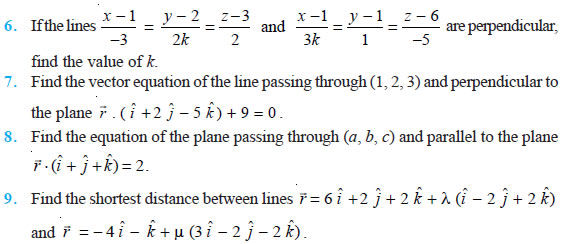Question 10. Find the coordinates of the point where the line through (5, 1, 6) and (3, 4,1) crosses the YZ-plane.

Question 11. Find the coordinates of the point where the line through (5, 1, 6) and (3, 4, 1) crosses the ZX-plane.

Question 12. Find the coordinates of the point where the line through (3, – 4, – 5) and (2, – 3, 1) crosses the plane 2x + y + z = 7.

Question 13. Find the equation of the plane passing through the point (– 1, 3, 2) and perpendicular to each of the planes x + 2y + 3z = 5 and 3x + 3y + z = 0.

Question 14. If the points (1, 1, p) and (– 3, 0, 1) be equidistant from the plane (3 ˆ + 4 ˆ −12 ˆ) +13 = 0, r i j k then find the value of p.

Question 15. Find the equation of the plane passing through the line of intersection of the planes r (iˆ + ˆj + kˆ) =1  and r (2 iˆ + 3 ˆj − kˆ) + 4 = 0 and parallel to x-axis.

Question 16. If O be the origin and the coordinates of P be (1, 2, – 3), then find the equation of the plane passing through P and perpendicular to OP.

Question 17. Find the equation of the plane which contains the line of intersection of the planes r (iˆ + 2 ˆj + 3 kˆ) − 4 = 0 , r (2 iˆ + ˆj − kˆ) + 5 = 0  and which is perpendicular to the plane r (5 iˆ + 3 ˆj − 6kˆ) + 8 = 0 ] .

Question 18. Find the distance of the point (– 1, – 5, – 10) from the point of intersection of the line r = 2 iˆ − ˆj + 2 kˆ + λ (3 iˆ + 4 ˆj + 2 kˆ)  and the plane r (iˆ − ˆj + kˆ) = 5 .

Question 19. Find the vector equation of the line passing through (1, 2, 3) and parallel to the planes r (iˆ − ˆj + 2kˆ) = 5  and r (3 iˆ + ˆj + kˆ) = 6 .

Question 20. Find the vector equation of the line passing through the point (1, 2, – 4) and perpendicular to the two lines: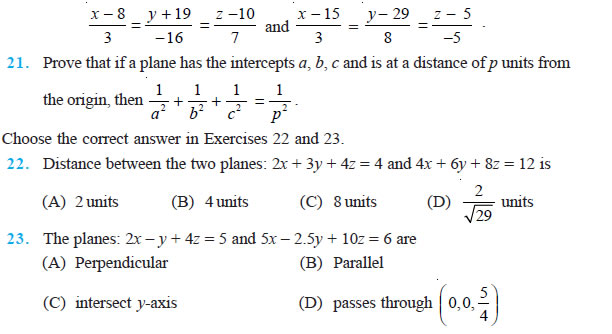### NCERT Books Free Pdf Download for Class 5, 6, 7, 8, 9, 10 , 11, 12 Hindi and English Medium

 Mathematics Biology Psychology Chemistry English Economics Sociology Hindi Business Studies Geography Science Political Science Statistics Physics Accountancy